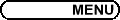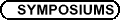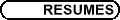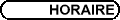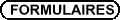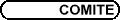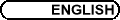Qualitative Behaviour and Controllability of Partial Differential Equations / Comportement qualitatif et controlabilité des EDP

DAVID AMUNDSEN, Carleton University
Resonant Solutions of the Forced KdV Equation

The forced Korteweg-de Vries (fKdV) Equation provides a canonical model for evolution of weakly nonlinear dispersive waves in the presence of additional effects such as external forcing or variable topography. While the symmetries and integrability of the underlying KdV structure facilitate extensive analysis, in this generalized setting such favourable properties no longer hold. Through physical and numerical experimentation it is known that a rich family of resonant steady solutions exist, yet qualitative analytic insight into them is limited. Based on hierarchical perturbative and matched asymptotic approaches we present a formal mathematical framework for construction of solutions in the small dispersion limit. In this way not only obtaining accurate analytic representations but also important a priori insight into the response of the system as it is detuned away from resonance. Specific examples and comparisons in the case of a fundamental periodic resonant mode will be presented.

Joint work with M. P. Mortell (UC Cork) and E. A. Cox (UC Dublin).

SEAN BOHUN, Penn State
The Wigner-Poisson System with an External Coulomb Field

This system of equations describes the time evolution of the quantum mechanical behaviour of a large ensemble of particles in a vacuum where the long range interactions between the particles can be taken into account. The model also facilitates the introduction of external classical effects. As tunneling effects become more pronounced in semiconductor devices, models which are able to bridge the gap between the quantum behaviour and external classical effects become increasingly relevant. The WP system is such a model.

Local existence is shown by a contraction mapping argument which is then extended to a global result using macroscopic control (conservation of probability and energy). Asymptotic behaviour of the WP system and the underlying SP system is established with a priori estimates on the spatial moments.

Finally, conditions on the energy are given which

(a) ensure that the solutions decay and
(b) ensure that the solutions do not decay.

SHAOHUA CHEN, University College of Cape Breton
Boundedness and Blowup for the Solution of an Activator-Inhibitor Model

We consider a general activator-inhibitor model

ì
ï
ï
í
ï
ï
î
 ut = eDu - mu + up vq ,
 vt = D Dv - nv + ur vs
with the Neumann boundary conditions, where rq > (p-1)(s+1). We show that if r > p-1 then the solutions exist long time for all initial values and if r > p-1 and q < s+1 then the solutions are bounded for all initial values. However, if r < p-1 then, for some special initial values, the solutions will blow up.

STEPHEN GUSTAFSON, University of British Columbia, Mathematics Department, 1984 Mathematics Rd., Vancouver, BC V6T 1Z2
Scattering for the Gross-Pitaevskii Equation

The Gross-Pitaevskii equation, a nonlinear Schroedinger equation with non-zero boundary conditions, models superfluids and Bose-Einstein condensates. Recent mathematical work has focused on the finite-time dynamics of vortex solutions, and existence of vortex-pair traveling waves. However, little seems to be known about the long-time behaviour (eg. scattering theory, and the asymptotic stability of vortices). We address the simplest such problem-scattering around the vacuum state-which is already tricky due to the non-self-adjointness of the linearized operator, and "long-range" nonlinearity. In particular, our present methods are limited to higher dimensions. This is joint work in progress with K. Nakanishi and T.-P. Tsai.

HORST LANGE, Universitaet Köln, Weyertal 86-90, 50931 Köln, Germany
Noncontrollability of the nonlinear Hartree-Schrödinger and Gross-Pitaevskii-Schrödinger equations

We consider the bilinear control problem for the nonlinear Hartree-Schrödinger equation [HS] (which plays a prominent role in quantum chemistry), and for the Gross-Pitaevskii-Schrödinger equation [GPS] (of the theory of Bose-Einstein condensates); for both systems we study the case of a bilinear control term involving the position operator or the momentum operator. A target state uT Î L2(R3) is said to be reachable from an initial state u0 Î L2(R3) in time T > 0 if there exists a control s.t. the system allows a solution state u(t,x) with u(0,x) = u0(x), u(T,x) = uT(x). We prove that, for any T > 0 and any initial datum u0 Î L2 (R3) \{0}, the set of non-reachable target states (in time T > 0) is relatively L2-dense in the sphere {u Î L2(R3) | ||u||L2 = ||u0||L2} (for both [HS] and [GPS]). The proof uses Fourier transform, estimates for Riesz potentials for [HS], estimates for the Schrödinger group associated with the Hamiltonian -D+x2 for [GPS].

HAILIANG LI, Department of Pure and Applied Mathematics, Osaka University, Japan
On Well-posedness and Asymptotics of Multi-dimensional Quantum Hydrodynamics

In the modelling of semiconductor devices in nano-size, for instance, MOSFET's and RTD's where quantum effects (like particle tunnelling through potential barriers and built-up in quantum wells) take place, the quantum hydrodynamical equations are important and dominative in the description of the motion of electron or hole transport under the self-consistent electric field. These quantum hydrodynamic equations consist of conservation laws of mass, balance laws of momentum forced by an additional nonlinear dispersion (caused by the quantum (Bohm) potential), and self-consistent electric field.

In this talk, we shall review the recent progress on the multi-dimensional quantum hydrodynamic equations, including the mathematical modelings based on the moment method applied to the Wigner-Boltzmann equation, rigorous analysis on the well-posedness for general, nonconvex pressure-density relation and regular large initial data, long time stability of steady-state under a quantum subsonic condition, and global-in-time relaxation limit from the quantum hydrodynamic equations to the quantum drift-diffusion equations, and so on.

Joint with A. Jüngel, P. Marcati, and A. Matsumura.

DONG LIANG, York University, 4700 Keele Street, Toronto, Ontario M3J 1P3
Analysis of the S-FDTD Method for Three-Dimensional Maxwell Equations

The finite-difference time-domain (FDTD) method for Maxwell's equations, firstly introduced by Yee, is a very popular numerical algorithm in computational electromagnetics. However, the traditional FDTD scheme is only conditionally stable. The computation of three-dimensional problems by the scheme will need much more computer memory or become extremely difficult when the size of spatial steps becomes very small. Recently, there is considerable interest in developing efficient schemes for the problems.

In this talk, we will present a new splitting finite-difference time-domain scheme (S-FDTD) for the general three-dimensional Maxwell's equations. Unconditional stability and convergence are proved for the scheme by using the energy method. The technique of reducing perturbation error is further used to derive a high order scheme. Numerical results are given to illustrate the performance of the methods.

This research is joint work with L. P. Gao and B. Zhang.

KIRSTEN MORRIS, University of Waterloo
Controller Design for Partial Differential Equations

Many controller design problems of practical interest involve systems modelled by partial differential equations. Typically a numerical approximation is used at some stage in controller design. However, not every scheme that is suitable for simulation is suitable for controller design. Misleading results may be obtained if care is not taken in selecting a scheme. Sufficient conditions for a scheme to be suitable for linear quadratic or H¥ controller design have been obtained. Once a scheme is chosen, the resulting approximation will in general be a large system of ordinary differential equations. Standard control algorithms are only suitable for systems with model order less than 100 and special techniques are required.

KEITH PROMISLOW, Michigan State University
Nonlocal Models of Membrane Hydration in PEM Fuel Cells

Polymer electrolyte membrane (PEM) fuel cells are unique energy conversion devices, effeciently generating useful electric voltage from chemical reactants without combustion. They have recently captured public attention for automotive applications for which they promise high performance without the pollutants associated with combustion.

>From a mathematical point of view the device is governed by coupled systems of elliptic, parabolic, and degenerate parabolic equations describing the heat, mass, and ion tranpsort through porous medias and polymer electrolyte membranes. This talk will describe the overall funtionality of the PEM fuel cell, presenting analysis of the slow, nonlocal propagation of hydration fronts within the polymer electrolyte membrane.

TAI-PENG TSAI, University of British Columbia, Vancouver
Boundary regularity criteria for suitable weak solutions of Navier-Stokes equations

I will present some new regularity criteria for suitable weak solutions of the Navier-Stokes equations near boundary in space dimension 3. Partial regularity is also analyzed.

This is joint work with Stephen Gustafson and Kyungkeun Kang.